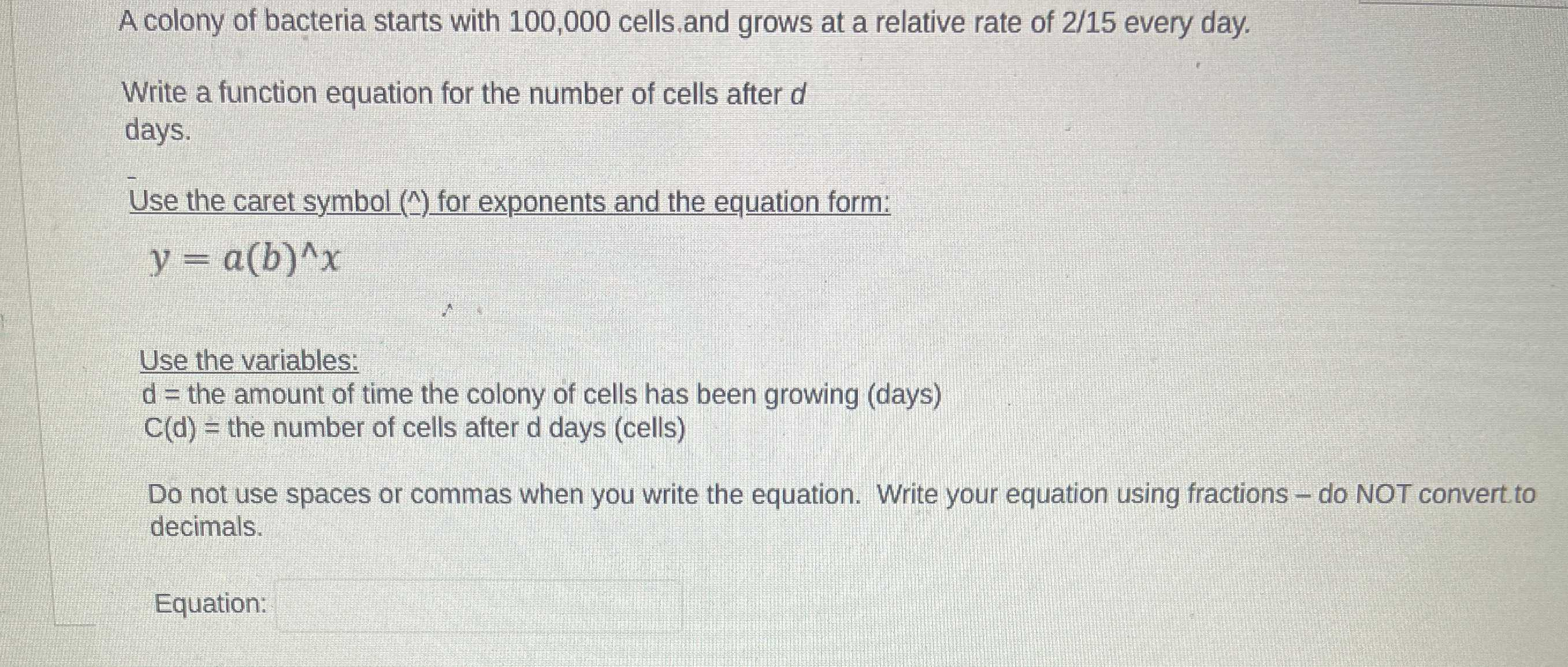### ¿Todavía tienes preguntas de matemáticas?

Pregunte a nuestros tutores expertos
Algebra
PreguntaA colony of bacteria starts with $$100,000$$ cells. and grows at a relative rate of $$2 / 15$$ every day. Write a function equation for the number of cells after $$d$$ days. Use the caret symbol (^) for exponents and the equation form: $$y = a ( b ) ^ { \wedge } x$$ Use the variables: $$d =$$ the amount of time the colony of cells has been growing (days) , $$C ( d ) =$$ the number of cells after d days (cells) Do not use spaces or commas when you write the equation. Write your equation using fractions - do NOT convert to decimals.

y=100000^($$\frac{2}{15}$$x)